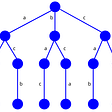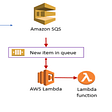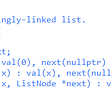# Leetcode

1196. How Many Apples Can You Put into the Basket

You have some apples, where `arr[i]` is the weight of the `i`-th apple. You also have a basket that can carry up to `5000` units of weight.

Return the maximum number of apples you can put in the basket.

Example 1:

`Input: arr = [100,200,150,1000]Output: 4Explanation: All 4 apples can be carried by the basket since their sum of weights is 1450.`

Example 2:

`Input: arr = [900,950,800,1000,700,800]Output: 5Explanation: The sum of weights of the 6 apples exceeds 5000 so we choose any 5 of them.`

Constraints:

• `1 <= arr.length <= 10^3`
• `1 <= arr[i] <= 10^3`

Solution:

`# min-heapclass Solution:    def maxNumberOfApples(self, arr: List[int]) -> int:        heapq.heapify(arr)        apples = units = 0        # arr always represents the smallest element in the min-heap        while arr and units + arr <= 5000:            units += heapq.heappop(arr)            apples += 1        return applesTC: O(N + k*longN), # k is the number of apples would put in the basketSC: O(1)# sortclass Solution:    def maxNumberOfApples(self, arr: List[int]) -> int:        arr.sort()        apples = units = 0        for _, weight in enumerate(arr):            units += weight            if units > 5000:                break            apples += 1         return applesTC: O(nlogn) # becoz of sortingSC: O(1)`

--

--

--

## More from JEN-LI CHEN IN DATA SCIENCE

My homepage to record my thought processes for solving SQL and Algorithm questions

## Squirrel Weekly (08/12) 🐿️## Backtracking Algorithm## Engineering## Using an Amazon Simple Queue Service to invoke a Lambda function on LocalStack## My First HTML & CSS Challenge## Algorithms and Data Structures Part VIII## Isabelle

In love with telling stories with data

## Linked List | LeetCode Top Interview Questions